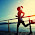## Tuesday, June 26, 2012

### MySQL Spatial Query Example

CREATE TABLE Points (
NAME VARCHAR(16) PRIMARY KEY,
location POINT NOT NULL,
description VARCHAR(128),
SPATIAL INDEX(location)
) ENGINE = MYISAM;

INSERT INTO Points (NAME, location) VALUES ( 'point1' , GEOMFROMTEXT( ' POINT(31.5 42.2) ' ) );
INSERT INTO Points (NAME, location) VALUES ( 'point2' , GEOMFROMTEXT( ' POINT(10 10) ' ) );
INSERT INTO Points (NAME, location) VALUES ( 'point3' , GEOMFROMTEXT( ' POINT(20 10) ' ) );
INSERT INTO Points (NAME, location) VALUES ( 'point4' , GEOMFROMTEXT( ' POINT(30 10) ' ) );
INSERT INTO Points (NAME, location) VALUES ( 'point5' , GEOMFROMTEXT( ' POINT(40 10) ' ) );
INSERT INTO Points (NAME, location) VALUES ( 'point6' , GEOMFROMTEXT( ' POINT(10 20) ' ) );
INSERT INTO Points (NAME, location) VALUES ( 'point7' , GEOMFROMTEXT( ' POINT(20 20) ' ) );
INSERT INTO Points (NAME, location) VALUES ( 'point8' , GEOMFROMTEXT( ' POINT(30 20) ' ) );
INSERT INTO Points (NAME, location) VALUES ( 'point9' , GEOMFROMTEXT( ' POINT(40 20) ' ) );
INSERT INTO Points (NAME, location) VALUES ( 'point10' , GEOMFROMTEXT( ' POINT(10 30) ' ) );
INSERT INTO Points (NAME, location) VALUES ( 'point11' , GEOMFROMTEXT( ' POINT(20 30) ' ) );
INSERT INTO Points (NAME, location) VALUES ( 'point12' , GEOMFROMTEXT( ' POINT(30 30) ' ) );
INSERT INTO Points (NAME, location) VALUES ( 'point13' , GEOMFROMTEXT( ' POINT(40 30) ' ) );
INSERT INTO Points (NAME, location) VALUES ( 'point14' , GEOMFROMTEXT( ' POINT(10 40) ' ) );
INSERT INTO Points (NAME, location) VALUES ( 'point15' , GEOMFROMTEXT( ' POINT(20 40) ' ) );
INSERT INTO Points (NAME, location) VALUES ( 'point16' , GEOMFROMTEXT( ' POINT(30 40) ' ) );
INSERT INTO Points (NAME, location) VALUES ( 'point17' , GEOMFROMTEXT( ' POINT(40 40) ' ) );
INSERT INTO Points (NAME, location) VALUES ( 'point18' , GEOMFROMTEXT( ' POINT(10 50) ' ) );
INSERT INTO Points (NAME, location) VALUES ( 'point19' , GEOMFROMTEXT( ' POINT(20 50) ' ) );
INSERT INTO Points (NAME, location) VALUES ( 'point20' , GEOMFROMTEXT( ' POINT(30 50) ' ) );
INSERT INTO Points (NAME, location) VALUES ( 'point21' , GEOMFROMTEXT( ' POINT(40 50) ' ) );
INSERT INTO Points (NAME, location) VALUES ( 'point22' , GEOMFROMTEXT( ' POINT(10 60) ' ) );
INSERT INTO Points (NAME, location) VALUES ( 'point23' , GEOMFROMTEXT( ' POINT(20 60) ' ) );
INSERT INTO Points (NAME, location) VALUES ( 'point24' , GEOMFROMTEXT( ' POINT(30 60) ' ) );
INSERT INTO Points (NAME, location) VALUES ( 'point25' , GEOMFROMTEXT( ' POINT(40 60) ' ) );

SELECT NAME, ASTEXT(location) FROM Points;

Query by Radius (10):

SET @center = GEOMFROMTEXT('POINT(25 25)');
SET @radius = 10;
SET @bbox = CONCAT('POLYGON((',
X(@center) - @radius, ' ', Y(@center) - @radius, ',',
X(@center) + @radius, ' ', Y(@center) - @radius, ',',
X(@center) + @radius, ' ', Y(@center) + @radius, ',',
X(@center) - @radius, ' ', Y(@center) + @radius, ',',
X(@center) - @radius, ' ', Y(@center) - @radius, '))'
);

SELECT NAME, ASTEXT(location)
FROM Points
WHERE INTERSECTS( location, GEOMFROMTEXT(@bbox) )
AND SQRT(POW( ABS( X(location) - X(@center)), 2) + POW( ABS(Y(location) - Y(@center)), 2 )) < @radius;

SELECT NAME, ASTEXT(location), SQRT(POW( ABS( X(location) - X(@center)), 2) + POW( ABS(Y(location) - Y(@center)), 2 )) AS distance
FROM Points
WHERE INTERSECTS( location, GEOMFROMTEXT(@bbox) )
AND SQRT(POW( ABS( X(location) - X(@center)), 2) + POW( ABS(Y(location) - Y(@center)), 2 )) < @radius
ORDER BY distance;

#### 1 comment:

1.